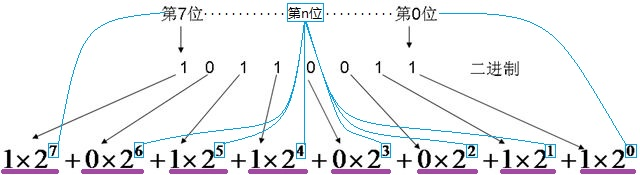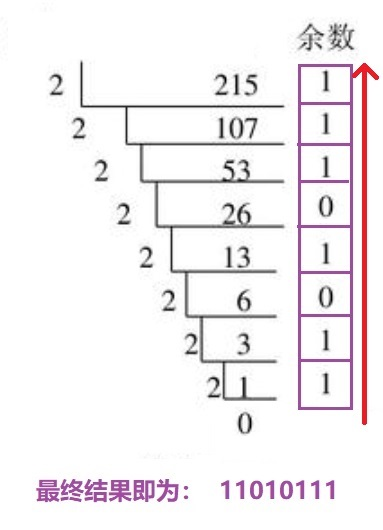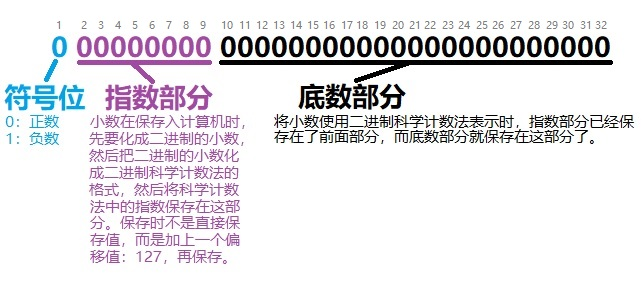## 【二进制,JAVA】二进制的认识和Java中对二进制的使用

byMicroanswer 创建时间：Aug 6, 2019 1:11:02 PM

### 二、位 - bit

• byte: 由8个位组成。
• short: 由16个位组成
• char: 由16个位组成
• int: 由32个位组成
• float: 由32个位组成
• long: 由64个位组成
• double: 由64个位组成

``````使用byte作为案例，因为只有8位，篇幅占用少。

00000010: 表示整数 2
11111110: 表示整数 -2。
取值过程：
第一步：观察最高位是1，因此取反，得：00000001
第二步：取反后加1，得：00000010
得出结果：2，取负得：-2。

0000 0010 = 2
1111 1110 = -2
``````

#### 1、二进制转十进制

``````十进制的基数是：0, 1, 2, 3, 4, 5, 6, 7, 8, 9

1  ==   1
10  ==   2
11  ==   3
100  ==   4
101  ==   5
110  ==   6
111  ==   7
1000  ==   8
1001  ==   9
1010  ==  10
1011  ==  11
1100  ==  12
1101  ==  13
1110  ==  14
1111  ==  15
``````#### 2、十进制转二进制### 三、字节 - byte

``````一个byte占8位，而最高位0表示正数，1表示负数。

1、最高位是1，为负数，先取反得：0111 1111；
2、再加1得：1000 0000;
3、使用上面的公式计算：1x(2^7)+0x（2^6）+...+0x(2^0) = 128
4、由于最高位是1，表示负数，得到最终结果：-128

1、直接使用公式计算：1x(2^6)+1x(2^5)+...+1x(2^0) = 127
2、由于最高位是0，直接确定最终结果：127

``````

### 七、浮点

#### 1、十进制小数与二进制的互换

##### 1)、十进制->二进制

``````// 只对小数部分乘2取整。
0.13 × 2 = 0.26 -> 取整数部分得：0 ，下次计算只使用小数部分：0.26
0.26 × 2 = 0.52 -> 取整数部分得：0 ，下次计算只使用小数部分：0.52
0.52 × 2 = 1.04 -> 取整数部分得：1 ，下次计算只使用小数部分：0.04
0.04 × 2 = 0.08 -> 取整数部分得：0 ，下次计算只使用小数部分：0.08
0.08 × 2 = 0.16 -> 取整数部分得：0 ，下次计算只使用小数部分：0.16
0.16 × 2 = 0.32 -> 取整数部分得：0 ，下次计算只使用小数部分：0.32
0.32 × 2 = 0.64 -> 取整数部分得：0 ，下次计算只使用小数部分：0.64
0.64 × 2 = 1.28 -> 取整数部分得：1 ，下次计算只使用小数部分：0.28
0.28 × 2 = 0.56 -> 取整数部分得：0 ，下次计算只使用小数部分：0.56
0.56 × 2 = 1.12 -> 取整数部分得：1 ，下次计算只使用小数部分：0.12
0.12 × 2 = 0.24 -> 取整数部分得：0 ，下次计算只使用小数部分：0.24
0.24 × 2 = 0.48 -> 取整数部分得：0 ，下次计算只使用小数部分：0.48
0.48 × 2 = 0.96 -> 取整数部分得：0 ，下次计算只使用小数部分：0.96
0.96 × 2 = 1.92 -> 取整数部分得：1 ，下次计算只使用小数部分：0.92
0.92 × 2 = 1.84 -> 取整数部分得：1 ，下次计算只使用小数部分：0.84
0.84 × 2 = 1.68 -> 取整数部分得：1 ，下次计算只使用小数部分：0.68
0.68 × 2 = 1.36 -> 取整数部分得：1 ，下次计算只使用小数部分：0.36
0.36 × 2 = 0.72 -> 取整数部分得：0 ，下次计算只使用小数部分：0.72
0.72 × 2 = 1.44 -> 取整数部分得：1 ，下次计算只使用小数部分：0.44
0.44 × 2 = 0.88 -> 取整数部分得：0 ，下次计算只使用小数部分：0.88
0.88 × 2 = 1.76 -> 取整数部分得：1 ，下次计算只使用小数部分：0.76
0.76 × 2 = 1.52 -> 取整数部分得：1 ，下次计算只使用小数部分：0.52
0.52 × 2 = ....... 发现又一次出现0.52，这和第4行的产生了重复，继续乘下去也会一直这样下去，这里就产生了二进制上的循环。

0.13 转化为二进制为：00 10000101000111101011 10000101000111101011....循环
``````

``````24.13 = 11000.00 10000101000111101011 10000101000111101011....循环
``````
##### 2)、二进制->十进制

``````11000 = 1×(2^4)+1×(2^3)+0×(2^2)+0×(2^1)+0×(2^0) = 24
``````

``````00 10000101000111101011 10000101000111101011....循环 = 0×(2^-1)+0×(2^-2)+1×(2^-3)+0×(2^-4)+0×(2^-5)+0×(2^-6)+0×(2^-7)+1×(2^-8)++0×(2^-9)+1×(2^-10)+0×(2^-11).... ≈0.13
``````

``````11000.00 10000101000111101011....循环 = 1×(2^4)+1×(2^3)+0×(2^2)+0×(2^1)+0×(2^0)+0×(2^-1)+0×(2^-2)+1×(2^-3)+0×(2^-4)+0×(2^-5)+0×(2^-6)+0×(2^-7)+1×(2^-8)++0×(2^-9)+1×(2^-10)+0×(2^-11).... ≈ 24.13
``````

#### 2、单精度浮点 - float

##### IEEE 754 标准

IEEE二进制浮点数算术标准（IEEE 754）是20世纪80年代以来最广泛使用的浮点数运算标准，为许多CPU与浮点运算器所采用。这个标准定义了表示浮点数的格式（包括负零-0）与反常值（denormal number）），一些特殊数值（无穷（Inf）与非数值（NaN）），以及这些数值的“浮点数运算符”；它也指明了四种数值舍入规则和五种例外状况（包括例外发生的时机与处理方式）。了解更多``````比如我们将小数 13.625 的指数部分要保存的值求出来：

要注意这个过程有个规定：化为科学计数法时必须要保证小数点左边只有一个数并且这个数只能是1。
因此这里我们只需要将 1101.101 向右移动(即小数点向左)3个位置，就可得到结果：1.101101 × 2^3

``````

``````接上一部分推导。我们已经拿到了 13.625 的二进制科学计数表示结果：1.101101 × 2^3

``````

``````小数：13.625

``````

#### 3、单精度浮点 - float - 取值范围

``````先计算最小值：1 11111111 11111111111111111111111

将二进制数：11111111 转化为十进制得：255
由于存入时的指数是加了偏移127的，因此取出时要减去，得： 255-127 = 128

由于底数存储是使用的小数点右边部分，因此取出是要加上原本左边的[1.]。
得到结果：1.11111111111111111111111

将底数和指数结合，得：1.11111111111111111111111 × 2^(128)

得到：1 11111111111111111111111 00...00 (除第一个1外，有23个1。共104个0)

1×(2^127) + 1×(2^126) + ... + 1×(2^104) + 0×(2^103) + ... + 0×(2^0)
= 340282346638528859811704183484516925440

-340282346638528859811704183484516925440 ~ 340282346638528859811704183484516925440
``````

### 八、字符 - char

0\t9\n10
\r13空格32
!33"34#35
\$36%37&38
'39(40)41
*42+43,44
-45.46/47
048149250
351452553
654755856
957:58;59
<60=61>62
?63@64A65
B66C67D68
E69F70G71
H72I73J74
K75L76M77
N78O79P80
Q81R82S83
T84U85V86
W87X88Y89
Z90[91|92
]93^94_95
`96a97b98
c99d100e101
f102g103h104
i105j106k107
l108m109n110
o111p112q113
r114s115t116
u117v118w119
x120y121z122
{123|124}125
~126

``````for (char i = 0; i < Character.MAX_VALUE; i++) {
System.out.println(i + ":" + (short)i);
}
``````